×#### Thank you for registering.

One of our academic counsellors will contact you within 1 working day.

Click to Chat

1800-1023-196

+91-120-4616500

CART 0

• 0

MY CART (5)

Use Coupon: CART20 and get 20% off on all online Study Material

ITEM
DETAILS
MRP
DISCOUNT
FINAL PRICE
Total Price: Rs.

There are no items in this cart.
Continue Shopping• Complete JEE Main/Advanced Course and Test Series
• OFFERED PRICE: Rs. 15,900
• View Details

```Chapter 11: Constructions​ Exercise – 11.3

Question: 1

Draw a circle of radius 6 cm. From a point 10 cm away from its center, construct a pair of tangents to the circle and measure their lengths.

Solution:

Given that: Construct a circle of radius 6 cm, and let a point P = 10 cm from its centre, construct a pair of tangents to the circle. Find the length of the tangents. We follow the following steps to construct the given: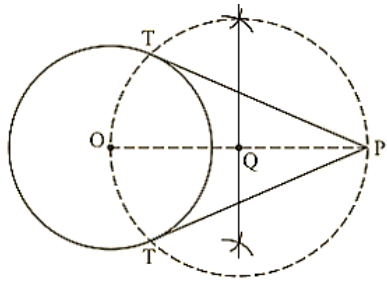Steps of construction:

1. First of all, we draw a circle of radius AB = 6 cm.

2. Make a point P at a distance of OP = 10 cm, and join OP.

3. Draw a right bisector of P, intersecting OP at Q.

4. Taking Q as center and radius OQ = PQ, draw a circle to intersect the given circle at T and T`.

5. Join PT and P`T` to obtain the required tangents. Thus, PT and P`T` are the required tangents. Find the length of the tangents.

As we know that OT⊥PT and ΔOPT is the right triangle.

Therefore, OT = 6 cm and PO = 10 cm.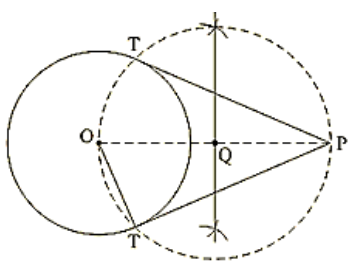In ΔOPT,

PT2 = OP2 − OT2

= (10)2 − (6)2

= 100 - 36

= 64 PT

= 8 cm

Thus, length of tangents = 8 cm.

​Question: 2

Draw a circle of radius 3 cm. Take two points P and Q on one of its extended diameter each at a distance of 7 cm from its center. Draw tangents to the circle from these points P and Q.

Solution:Steps of construction:

1. Draw a line segment PQ of 14 cm.

2. Take the midpoint O of PQ.

3. Draw the perpendicular bisectors of PO and OQ which intersects at points R and S.

4. With center R and radius RP draw a circle.

5. With center S and radius, SQ draw a circle.

6. With center O and radius 3 cm draw another circle which intersects the previous circles at the points A, B, C, and D.

7. Join PA, PB, QC, and QD. So, PA, PB, QC, and QD are the required tangents.

​Question: 3

Draw a line segment AB of length 8 cm. Taking A as the center, draw a circle of radius 4 cm and taking B as the center, draw another circle of radius 3 cm. Construct tangents to each circle from the center of the other circle.

Solution: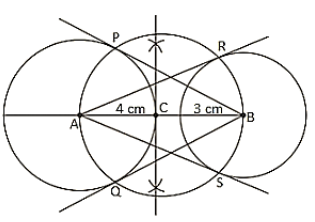Steps of construction:

1. Draw a line segment AB of 8 cm.

2. Draw the perpendicular of AB which intersects it at C.

3. With the center, C and radius CA draw a circle.

4. With centers A and B radius 4 cm and 3 cm, draw two circle which intersects the previous at the points P, Q, R and S.

5. Join AR, AS, BP and BQ So, AR, AS, BP and BQ are the required tangents.

​Question: 4

Draw two tangents to a circle of radius 3.5 cm from a point P at a distance of 6.2 cm from its center.

Solution: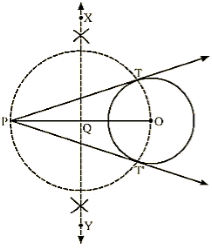Steps of construction:

1. Draw a circle with O as a center and radius 3.5 cm.

2. Mark a point P outside the circle such that OP = 6.2 cm

3. Join OP. Draw the perpendicular bisector XY of OP, cutting OP at Q.

4. Draw a circle with Q as center and radius PQ( or OQ), to intersect the given circle at the points T and T`.

5. Join PT and PT`.

Here, PT and PT` are the required tangents.

​Question: 5

Draw a pair of tangents to a circle of radius 4.5 cm, which are inclined to each other at an angle of 45°.

Solution: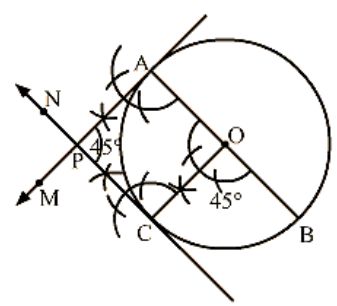Steps of Construction:

1. Draw a circle with center O and radius 4.5 cm.

2. Draw any diameter AOB of the circle.

3. Construct ∠BOC = 45∘ such that, radius OC cuts the circle at C.

4. Draw AM⊥ AB and CN⊥OC.

Suppose AM and CN intersect each other at P.

Here, AP and CP are the pairs of tangents to the circle inclined to each other at an angle of 45°.

​Question: 6

Draw a right triangle ABC in which AB = 6 cm, BC = 6 cm and ∠B = 90∘. Draw BD perpendicular from B on AC and draw a circle passing through the points B, C and D. Construct tangents from A to this circle.

Solution:

Steps of construction:

1. Draw a line segment AB = 6 cm

2. At B, draw ∠ABX = 90°.3.With B as center and radius 8 cm, draw an arc cutting ray BX at C.

4. Join AC. Thus, ΔABC is the required triangle.

5. From B, draw BD⊥ AC.

6. Draw the perpendicular bisector of BC, cutting BC at O.

7. With O as center and radius OB (or OC), draw a circle. This circle passes through B, C and D.

8.Thus, this is the required circle.

9. Join OA.

10. Draw the perpendicular bisector of OA, cutting OA at E.

11. With E as a center and radius AE (or OE), draw a circle intersecting the circle with center O at B and F.

12. Join AF.

Here, AB and AF are the required tangents.
```### Course Features

• 728 Video Lectures
• Revision Notes
• Previous Year Papers
• Mind Map
• Study Planner
• NCERT Solutions
• Discussion Forum
• Test paper with Video Solution